International
Tables for
Crystallography
Volume F
Crystallography of biological macromolecules
Edited by M. G. Rossmann and E. Arnold

International Tables for Crystallography (2006). Vol. F, ch. 12.2, pp. 256-257   | 1 | 2 |

Section 12.2.1. The origin of the phase problem

M. T. Stubbsa* and R. Huberb

aInstitut für Pharmazeutische Chemie der Philipps-Universität Marburg, Marbacher Weg 6, D-35032 Marburg, Germany, and bMax-Planck-Institut für Biochemie, 82152 Martinsried, Germany
Correspondence e-mail:  stubbs@mailer.uni-marburg.de

12.2.1. The origin of the phase problem

| top | pdf |

Once a native data set has been collected, the next task is the solution of the structure. There is one major hurdle: the phase problem. To study objects at the atomic level, we must utilize waves with a wavelength in the ångström range, i.e. X-radiation. X-rays interact with electrons and so provide an image of the electron distribution of the sample. Unfortunately, X-rays are refracted by matter only very weakly, and so it is not possible to construct a lens to view molecules at atomic dimensions.1

As shown in Chapter 2.1, the diffraction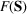obtained from an electron-density distributionis given by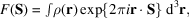where S is perpendicular to the scattered wave and; θ is the scattering angle and λ is the wavelength. The diffraction pattern is a Fourier transform of the electron density. If we have a crystal with cell parameters a, b and c, then the Laue diffraction conditions require that S lies on a reciprocal lattice such that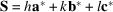, where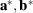andare the reciprocal-lattice vectors, and h, k and l are the integer indices of the diffracted beam.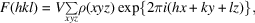where V represents the volume of the unit cell, and x, y and z are the fractional coordinates within that cell in the directions of a, b and c.

Since the diffraction pattern is a Fourier transform of the electron density, it follows that the electron density is an inverse Fourier transform of the diffraction pattern: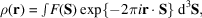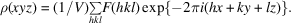Thus it should be mathematically straightforward to calculate the electron density from the diffraction pattern. This is, unfortunately, not the case. The functiondescribing the diffracted rays is a complex function with a magnitudeand a phase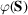. The diffraction experiment measures the intensities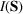, however; the relationship betweenandis: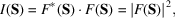where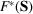is the complex conjugate of. The measured intensities are related directly to the magnitudes of the diffracted beams; the phase information, however, is lost (Fig. 12.2.1.1): this is the origin of the phase problem.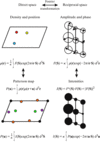Figure 12.2.1.1 | top | pdf |Relationships in diffraction space. The diffraction pattern of an object is the Fourier transform of the electron density, consisting of both amplitudes and phases. What we measure in an X-ray diffraction experiment, however, are the diffracted intensities; the phase information is lost. The Fourier transform of the intensities results in the Patterson map, which is related to the electron density as follows. For any two atoms in the structure, the vector between them, centred at the origin, has a value corresponding to the product of their densities. Thus, the red atom and the yellow atom result in the orange cross vectors, the red and blue atoms result in the magenta cross vectors, and the yellow and blue atoms result in green cross vectors. As each atom has a `cross vector' to itself, a large peak is found at the origin; the Patterson map is centrosymmetric.

There are essentially four ways of overcoming the phase problem (Fig. 12.2.1.2):

• (1) the use of isomorphous replacement to influence the diffraction pattern, thereby revealing information about the phases (this chapter);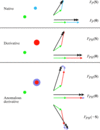Figure 12.2.1.2 | top | pdf |The effect of introducing a heavy atom or anomalous scatterer. The native two-atom structure gives rise to two diffraction vectors (green and blue) of equal magnitude but different phase (see Chapter 2.1), with a resultant diffraction vector(black). Isomorphous replacement of the blue atom by the larger red one gives rise to a diffraction vector of greater magnitude but equivalent phase (red), causing a change in the resultant magnitude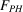(and hence the intensity) and in the phase. Introduction of an anomalous scatterer results in a phase shift (lilac) of the diffraction vector, resulting in differing amplitudes and phases for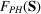and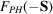.
• (2) Patterson search techniques, which in essence allow modelling of the phase distribution of an unknown crystal based upon that of a known molecule (Part 13);

• (3) the use of anomalous dispersion, which shifts the imaginary component of, allowing an experimental measurement of the phase (Part 14); and

• (4) direct methods, which make use of probabilistic relationships between different diffracted rays (Part 16).

The method of isomorphous replacement, by which the first macromolecular structures were solved (Green et al., 1954), remains the most widely used technique for ab initio structure determination, although the availability of synchrotrons, with their facility for selecting a desired wavelength, and molecular-biology techniques that allow the direct introduction of anomalous scatterers, such as selenium or tellurium, into the protein of interest (Hendrickson et al., 1990; Budisa et al., 1997) have proven that multiple anomalous dispersion is an exceptionally powerful technique for the solution of novel structures. Patterson search techniques (Rossmann, 1972) are ideal if a similar macromolecular structure is already known, while direct methods are more-or-less confined to very high resolution data (Sheldrick, 1990).

In order to obtain phase information from isomorphous replacement (or from anomalous dispersion), it is necessary to locate the atomic positions of the heavy-atom (or anomalous) scatterers.

References

Budisa, N., Karnbrock, W., Steinbacher, S., Humm, A., Prade, L., Neuefeind, T., Moroder, L. & Huber, R. (1997). Bioincorporation of telluromethionine into proteins: a promising new approach for X-ray structure analysis of proteins. J. Mol. Biol. 270, 616–623.Google Scholar
Green, D. W., Ingram, V. M. & Perutz, M. F. (1954). The structure of haemoglobin IV. Sign determination by the isomorphous replacement method. Proc. R. Soc. London Ser. A, 225, 287–307.Google Scholar
Hendrickson, W. A., Horton, J. R. & LeMaster, D. M. (1990). Selenomethionyl proteins produced for analysis by multiwavelength anomalous diffraction (MAD): a vehicle for direct determination of three-dimensional structure. EMBO J. 9, 1665–1672.Google Scholar
Rossmann, M. G. (1972). Editor. The molecular replacement method. New York: Gordon and Breach. Google Scholar
Sheldrick, G. M. (1990). Phase annealing in SHELX-90: direct methods for larger structures. Acta Cryst. A46, 467–473.Google Scholar